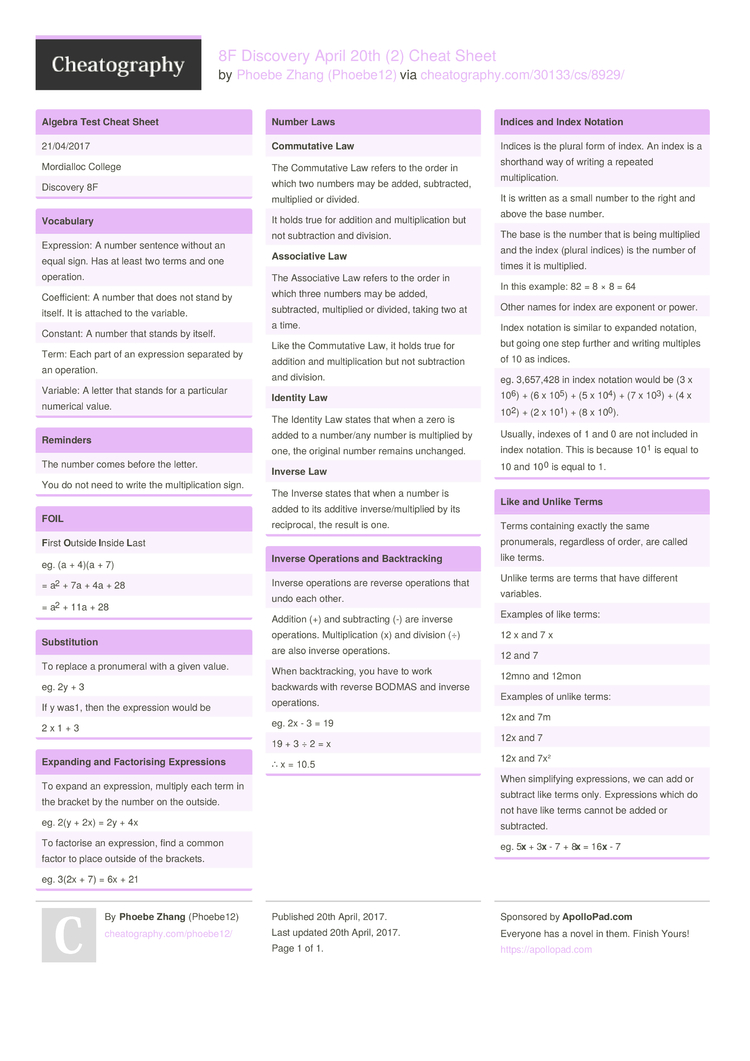# 8F Discovery April 20th (2) Cheat Sheet by Phoebe12

A cheat sheet for school.

### Algebra Test Cheat Sheet

 21/04/2017 Mordialloc College Discovery 8F

### Vocabulary

 Expres­sion: A number sentence without an equal sign. Has at least two terms and one operation. Coeffi­cient: A number that does not stand by itself. It is attached to the variable. Constant: A number that stands by itself. Term: Each part of an expression separated by an operation. Variable: A letter that stands for a particular numerical value.

### Reminders

 The number comes before the letter. You do not need to write the multip­lic­ation sign.

### FOIL

 First Ou­tside Inside Last eg. (a + 4)(a + 7) = a2 + 7a + 4a + 28 = a2 + 11a + 28

### Substi­tution

 To replace a pronumeral with a given value. eg. 2y + 3 If y was1, then the expression would be 2 x 1 + 3

### Expanding and Factor­ising Expres­sions

 To expand an expres­sion, multiply each term in the bracket by the number on the outside. eg. 2(y + 2x) = 2y + 4x To factorise an expres­­sion, find a common factor to place outside of the brackets. eg. 3(2x + 7) = 6x + 21

### Number Laws

 Comm­utative Law The Commut­ative Law refers to the order in which two numbers may be added, subtra­cted, multiplied or divided. It holds true for addition and multip­lic­ation but not subtra­ction and division. Asso­ciative Law The Associ­ative Law refers to the order in which three numbers may be added, subtra­cted, multiplied or divided, taking two at a time. Like the Commut­ative Law, it holds true for addition and multip­lic­ation but not subtra­ction and division. Identity Law The Identity Law states that when a zero is added to a number/any number is multiplied by one, the original number remains unchanged. Inverse Law The Inverse states that when a number is added to its additive invers­e/m­ult­iplied by its recipr­ocal, the result is one.

### Inverse Operations and Backtr­acking

 Inverse operations are reverse operations that undo each other. Addition (+) and subtra­cting (-) are inverse operat­ions. Multip­lic­ation (x) and division (÷) are also inverse operat­ions. When backtr­acking, you have to work backwards with reverse BODMAS and inverse operat­ions. eg. 2x - 3 = 19 19 + 3 ÷ 2 = x ∴ x = 10.5

### Indices and Index Notation

 Indices is the plural form of index. An index is a shorthand way of writing a repeated multip­lic­ation. It is written as a small number to the right and above the base number. The base is the number that is being multiplied and the index (plural indices) is the number of times it is multip­lied. In this example: 82 = 8 × 8 = 64 Other names for index are exponent or power. Index notation is similar to expanded notation, but going one step further and writing multiples of 10 as indices. eg. 3,657,428 in index notation would be (3 x 106) + (6 x 105) + (5 x 104) + (7 x 103) + (4 x 102) + (2 x 101) + (8 x 100). Usually, indexes of 1 and 0 are not included in index notation. This is because 101 is equal to 10 and 100 is equal to 1.

### Like and Unlike Terms

 Terms containing exactly the same pronum­erals, regardless of order, are called like terms. Unlike terms are terms that have different variables. Examples of like terms: 12 x and 7 x 12 and 7 12mno and 12mon Examples of unlike terms: 12x and 7m 12x and 7 12x and 7x² When simpli­fying expres­sions, we can add or subtract like terms only. Expres­sions which do not have like terms cannot be added or subtra­cted. eg. 5x + 3x - 7 + 8x = 16x - 71 Page
//media.cheatography.com/storage/thumb/phoebe12_8f-discovery-april-20th-2.750.jpg

PDF (recommended)